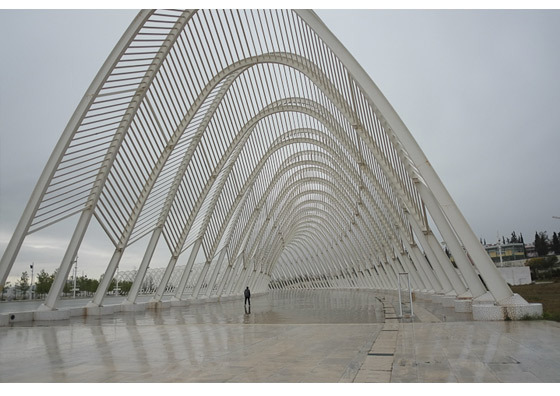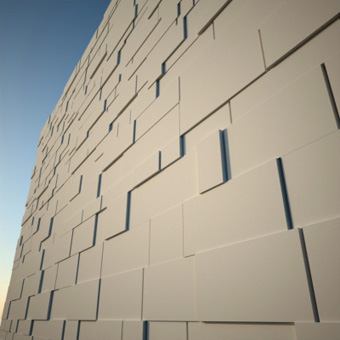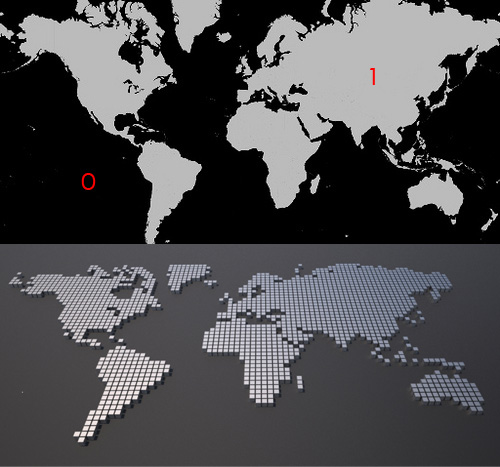Ali Torabi

Digital Architecture & Technology

Array on curves inside another arrayIn this video I’m creating a curve network. A curve network consist of a series of curves which has been assigned to curves from another array.
Also you learn how to use Xform modifier to transform selected vertices of a shape object.

PART I

PART II

, , , ,
June 12, 2012 at 11:55 pm Comments (0)

Parametric wall with PARA 2This is a new edition of old video tutorial (Parametric Brick wall) which uses link and math controllers to create a random pattern of stone blocks.

, , , , ,
March 11, 2012 at 10:11 am Comments (0)

Collect items of the array which satisfy your condition.In this video we use collection controller to find the position of those points which they are located on white sections of an image. The image is being process by a bitmap controller and if the value is greater than .5 (gray) the test controller returns true. collection controller goes through the entire array of points and collects only the position of the points which meets our condition. to set the position of each box to the corresponding point in collection we use a list controller. list controller takes the index value (location of the box in the array) and return the same item from the collection.

, , , , , , ,
March 1, 2012 at 11:27 am Comments (0)

Introducing intersection controller.

In first video you learn how to find the intersection of two coplanar lines. Notice that if two lines are not intersecting program will generates some error. in the second video we find the closest point on one line to a series of lines from another parametric array. Program automatically sets the controllers for point A and B. if two lines are actually intersection the closest point is equal to the intersection point. also you can find the apparent intersection of two lines on a plane. For example the apparent intersection on XY plane is the intersection of projected lines on this plane(intersection from top view) .

, , , , ,
February 28, 2012 at 11:24 am Comments (0)